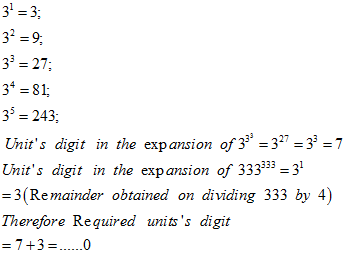• This is an assessment test.
• These tests focus on the basics of Maths and are meant to indicate your preparation level for the subject.
• Kindly take the tests in this series with a pre-defined schedule.

## Basic Maths: Test 37

Congratulations - you have completed Basic Maths: Test 37.

You scored %%SCORE%% out of %%TOTAL%%.

Your performance has been rated as %%RATING%%

 Question 1
The value of $\left( 1-\frac{1}{2} \right)\,\left( 1-\frac{1}{3} \right)\,\left( 1-\frac{1}{4} \right)\,.......\left( 1-\frac{1}{120} \right)$is
 A 1/120 B 120 C 119/120 D 1
Question 1 Explanation:
\begin{align} & \left( \frac{2-1}{2} \right)\,\left( \frac{3-1}{3} \right)\,\left( \frac{4-1}{4} \right)\,.......\left( \frac{120-1}{120} \right) \\ & =\frac{1}{2}\times \frac{2}{3}\times \frac{3}{4}\times ......\times \frac{119}{120} \\ & =\frac{1}{120} \\ \end{align}
 Question 2
The least on among the followingis
 A $13\frac{1}{2}%\,\,of\,\,100$ B $13.\overline{55}$ C ${{\left( \frac{18.5}{5} \right)}^{2}}$ D $\sqrt{187}$
Question 2 Explanation:
\begin{align} & 13\frac{1}{2}%\,\,of\,\,100=\frac{27}{2}=13.5 \\ & \\ & 13.\overline{55}=13\frac{55}{99}\simeq 13.555555556(approx) \\ & {{\left( \frac{18.5}{5} \right)}^{2}}={{\left( 3.7 \right)}^{2}}=13.69 \\ & =\sqrt{187}=13.675 \\ \end{align}
 Question 3
Which of the following is the largest?
 A 100010000 B 2100000 C 1000001000 D 3200
Question 3 Explanation:
\begin{align} & {{\left( 1000 \right)}^{10000}}={{\left( {{10}^{3}} \right)}^{10000}}={{\left( 10 \right)}^{30000}} \\ & {{\left( 100000 \right)}^{1000}}={{\left( {{10}^{5}} \right)}^{1000}}={{10}^{5000}} \\ & {{2}^{100000}}={{\left( {{2}^{10}} \right)}^{10000}}={{\left( 1024 \right)}^{10000}} \\ & {{\left( 1024 \right)}^{10000}}>{{\left( 1000 \right)}^{10000}} \\ & {{3}^{200}}={{\left( {{3}^{2}} \right)}^{100}}={{\left( 9 \right)}^{100}} \\ & {{\left( 9 \right)}^{100}}\le {{\left( 10 \right)}^{100}} \\ \end{align}
 Question 4
A sum of three non-zero numbers is five times the first number, four times the second number and k times the third number. The value of k is
 A 11/20 B 20/13 C 20/11 D 11/24
Question 4 Explanation:
\begin{align} & If\,\,the\,\,\,sum\,\,\,\,of\,\,\,three\,\,\,non-zero\,\,\,\operatorname{int}egers\,\,\,be\,\,\,S,\,\,then \\ & First\,\,\,number=\frac{S}{5} \\ & \operatorname{Sec}ond\,\,\,number=\frac{S}{4} \\ & Third\,\,\,number\,=\frac{S}{k} \\ & Therefore\,\,\,\frac{1}{5}+\frac{1}{4}+\frac{1}{k}=1 \\ & \Rightarrow \frac{1}{k}=1-\frac{1}{5}-\frac{1}{4}=\frac{20-4-5}{20} \\ & \Rightarrow \frac{1}{k}=\frac{11}{20} \\ & \Rightarrow k=\frac{20}{11} \\ \end{align}
 Question 5
Unit’s digit of $\left( {{3}^{\left( {{3}^{3}} \right)}}+{{333}^{333}} \right)$ is
 A 0 B 4 C 1 D 7
Question 5 Explanation:Once you are finished, click the button below. Any items you have not completed will be marked incorrect.
There are 5 questions to complete.
 ← List →
Shaded items are complete.
 1 2 3 4 5 End